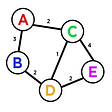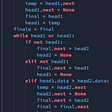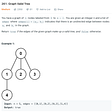# Parenthesis, Left, Right and Asterisk

1. a left or right parenthesis needs to be closed, like this:
• start from left, go through each character in the string.
• keep a count of left parenthesis, subtract 1 left parenthesis for every right parenthesis encountered.
• if left count dips below 0, the string is invalid. After going through the entire string, if the left count is exactly zero, then it’s a valid string.
`def check_valid_001(input):    left = 0    lp = '('    rp = ')'    for c in input:        if c is lp:            left = left + 1        elif c is rp:            left = left - 1        if left < 0:            return False    if left == 0:        return True    return False`
`def what_to_do(input):    left = 0    lp = '('    rp = ')'    asterisk = '*'    for c in input:        if c is lp:            left = left + 1        elif c is rp:            left = left - 1        elif c is asterisk:            # what to do?        if left < 0:            return False    if left == 0:        return True    return False`
`elif c is asterisk:    left = left + 1`
`elif c is asterisk:    left = left - 1`
`def check_valid_002(input):    left = 0    need_right = 0    lp = '('    rp = ')'    asterisk = '*'    for c in input:        if c is lp:            left = left + 1            need_right = need_right + 1        elif c is rp:            left = left - 1            need_right = need_right - 1        elif c is asterisk:            need_right = need_right - 1        if left < 0:            return False    if need_right == 0:        return True    return False`
`need_right = max(need_right, 0)`
`if left < 0:    return False`
`elif c is asterisk:    left = left + 1    need_right = need_right - 1`
`def check_valid_final(input):    left = 0    need_right = 0    lp = '('    rp = ')'    asterisk = '*'    for c in input:        if c is lp:            left = left + 1            need_right = need_right + 1        elif c is rp:            left = left - 1            need_right = need_right - 1        elif c is asterisk:            left = left + 1            need_right = need_right - 1        need_right = max(need_right, 0)        if left < 0:            return False    if need_right == 0:        return True    return Falsedef test(validator):    inputs = [        '())', '(())', '()()', '(()', '(()())',        '*', '**', '(*', '*)',        '(**', '**)', '*()', '((*'    ]    for input in inputs:        print(f'test {input} valid: {validator(input)}')# print('test 001')# test(check_valid_001)# print('test 002')# test(check_valid_002)print('test final')test(check_valid_final)`

--

--

--

## More from Shuo Wang

Interesting pieces on various topics in finance and technology.

Love podcasts or audiobooks? Learn on the go with our new app.

## Let’s Migrate Symfony Project to Kubernetes!## Deploy a containerised Node.js application to Cloud Run## ZALANDO LOGBOOK## Breaking the HTB Habits and building better OSCP ones…## Updates on Ethereum’s Moon Project## Becoming a software developer: How computer science is helping me becoming a better software…## Fast and reliable Persistent storage for Kubernetes with StorPool## {UPDATE} Barco 3D: Aventura en el Mar.## Shuo Wang

Interesting pieces on various topics in finance and technology.

## Graph Data Structures## Hackerrank — Merge Sorted Linked list walkthrough #Python #Hackerrank## Graph Valid Tree: Leetcode — Blind 75 (Python)